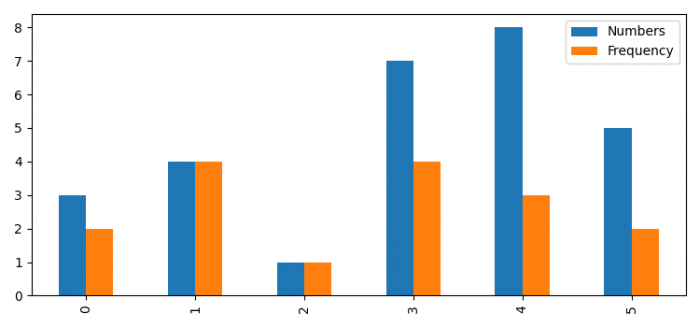# Create a legend with Pandas and Matplotlib.pyplot

MatplotlibPandasPythonData Visualization

#### Python Data Science basics with Numpy, Pandas and Matplotlib

Most Popular

63 Lectures 6 hours

#### Data Visualization using MatPlotLib & Seaborn

11 Lectures 4 hours

#### MatPlotLib with Python

9 Lectures 2.5 hours

To create a legend with Pandas and matplotib.pyplot(), we can take the following steps −

• Set the figure size and adjust the padding between and around the subplots.
• Make a two-dimensional, size-mutable, potentially heterogeneous tabular data.
• Plot the dataframe instance with bar class by name and legend is True.
• To display the figure, use show() method.

## Example

import pandas as pd
from matplotlib import pyplot as plt

plt.rcParams["figure.figsize"] = [7.50, 3.50]
plt.rcParams["figure.autolayout"] = True

fig, ax = plt.subplots()

df = pd.DataFrame({'Numbers': [3, 4, 1, 7, 8, 5], 'Frequency': [2, 4, 1, 4, 3, 2]})
df.plot(ax=ax, kind='bar', legend=True)

plt.show()

## Output